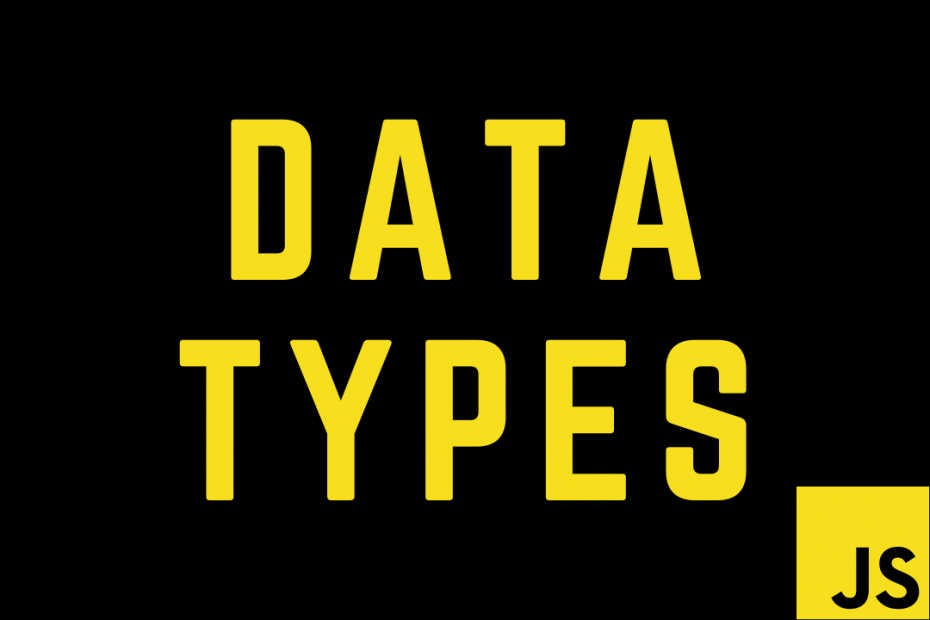# JavaScript Data Types 101

Data Type is the type/nature of data held in a variable. According to the latest ECMAScript standard definition, there are nine types of data in JavaScript. In this post, I’ll explain each of the data types with some examples.

## Dynamic Typing

Javascript is Dynamically Typed, also known as a Loosely Typed language. Meaning that we need not define the type of a variable and JavaScript dynamically assigns the type based on the data stored in it.

## Data Types

According to MDN, these are the following 9 types of data

Primitive data types

• String
• Boolean
• Number
• Undefined
• BigInt
• Symbol

Structural data types

• Object
• Function

Structural root primitive

• null

## Primitive data types

### String

The string is used to store text. We can define a string using single quotes, double quotes, and backticks. There is no difference between single and double quotes but, when we use backticks we can concatenate and add other variables/expressions inside a string.

``````const firstName = "Dexter";
const lastName = "Morgan";

const id = 77;
const work = `Analyst \${id}`;
console.log(work); // Analyst 77``````

### Boolean

The boolean represents logical values, it can either be `true` or `false`.

``````const isLoading = true;
const darkMode = false;``````

### Number

The number represents both integers and floating point number in JavaScript.

``````const age = 18;
const height = 155;``````

A number can aslo exist as `+Infinity`, `-Infinity`, and `NaN` in some cases.

`````` const division = 9 / +0;
console.log(division); // Infinity

const divide = 9 / -0;
console.log(divide); // -Infinity

const multiply = 9 * undefined;
console.log(multiply);  // NaN``````

### Safe limit for Numbers

The number stores double-precision 64-bit binary format IEEE 754 value (numbers between -(2^53 − 1) and (2^53 + 1). Any number greater than this is not safe and can result in approximate values or inconsistent results. In such a case, it is safe to use BigInt. We can alose use the method `Number.isSafeInteger()` to check whether a provided number is a safe integer, it returns a boolean value.

### Undefined

As suggested by the name undefined means that no value is assigned to a variable.

``````let count;
console.log(count); // undefined

// Moreover we can explicitly assign undefined
let name = undefined;``````

### BigInt

BigInt can be used to store numbers larger than the safe limit i.e (2**53 +/- 1). A BigInt is created by appending `n` to the end of an integer or by calling the constructor.

``const bigNumber = 1234567890123456789012345678901234567890n;``

### Symbol

The symbol data type was introduced in ES5 that is used to create unique values or identifiers. Every symbol is unique by default. Additionally, we can pass an optional description to the constructor.

``````const oneSymbol = Symbol('hello');
const twoSymbol = Symbol('hello');

oneSymbol === twoSymbol; // false``````

As we can see above both the symbols have the same description ‘hello’. However, they are unique, due to these properties symbols have a variety of use cases.

## Structural data types

### Object

Objects are used to store complex data types and collection of data. Data structures like Array, Set, HashMap, Date are of type object. Even functions are of type object.

``````const user = {
firstName: "Neo",
lastName: undefined,
age: 44,
colors: ["Red", "Blue"],
};``````

### Function

As stated above function is a type of object but, `typeof(function(){})` returns ‘function’. Because the function is an object that implements [[Call]].

``````function foo() {
console.log("hello world");
}``````

## Structural root primitive

### Null

The null is a primitive value due to its behavior. null generally means an unknown value, non-existent or wrong address.

``const name = null;``

null is of the type object this is a bug existing from the start of JavaScript and cannot be fixed now as it might break existing code (reference).

📝Note: All data types in JavaScript are immutable (values cannot be modified) except for the objects.

Tags: